# Chapter 10 Self Test

## CO/NO Problem

1)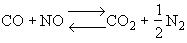Mechanism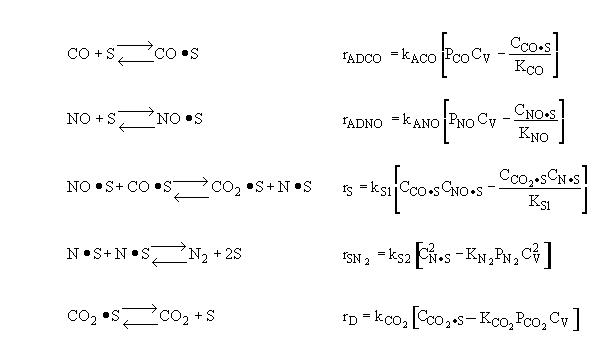Surface Reaction Limits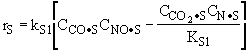Which of the following is false:

 A: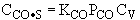B: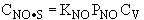C: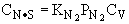D: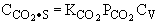E: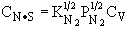2)Which of the following rate laws is correct

 A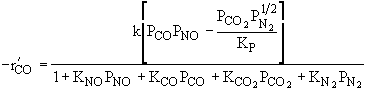B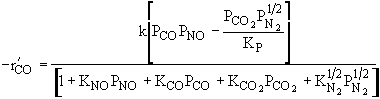C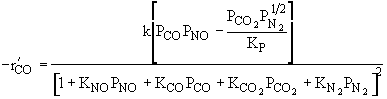D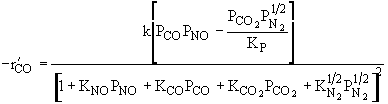3)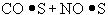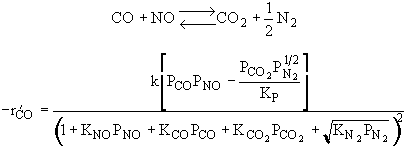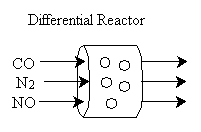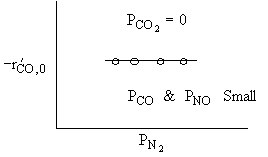What can you tell from the above figure? Reaction is irreversible. N2 is weakly adsorbed. NO is weakly adsorbed. Reaction is at equilibrium. None of the above.

4)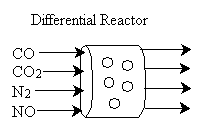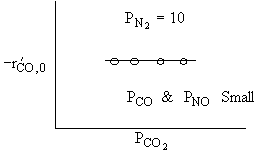Which of the following are true? Reaction is irreversible. CO2 is weakly adsorbed. Reaction is at equilibrium. CO is weakly adsorbed. NO is weakly adsorbed.

5)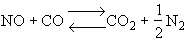For a fixed concentration of NO which of the following curves describes the rate law for the case when PNO is very very small?

 A B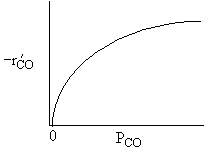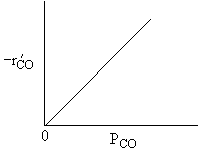C D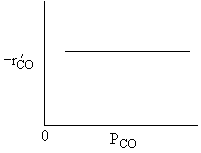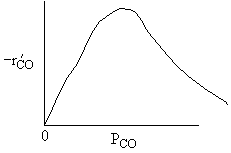E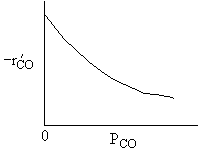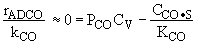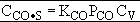A is True Similarly,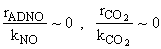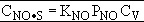B is True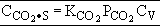D is True But,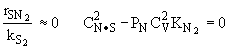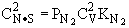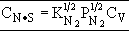E is True C is false

Back to Problem #1

Problem #2

Back to Chapter 10

 The rate law is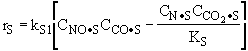and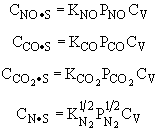Substituting for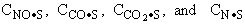in the rate law we obtain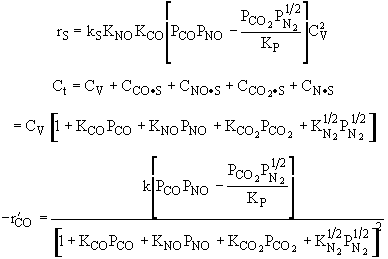Ans:  D

Problem #3

Back to Chapter 10Rate law suggests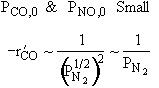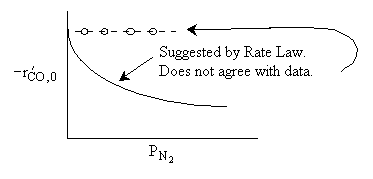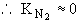Ans: B, N2 weakly adsorbed

Problem #4

Back to Chapter 10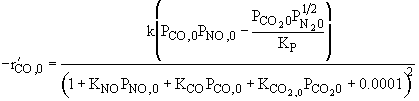1)      Reaction is irreversible.2)      CO2 is weakly adsorbed.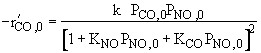1 and 2 are true, all others are false.

Back to Problem #4

Problem #5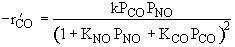Low Partial Pressure CO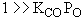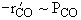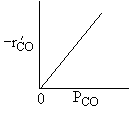High Partial Pressure CO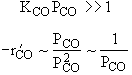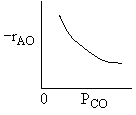Combining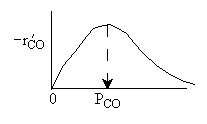Ans: DDifferentiating the rate law in with respect PCO to find the maximum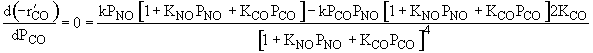Solving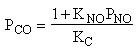Back to Chapter 10